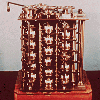You may also likeRoute to Root

A sequence of numbers x1, x2, x3, ... starts with x1 = 2, and, if you know any term xn, you can find the next term xn+1 using the formula: xn+1 = (xn + 3/xn)/2 . Calculate the first six terms of this sequence. What do you notice? Calculate a few more terms and find the squares of the terms. Can you prove that the special property you notice about this sequence will apply to all the later terms of the sequence? Write down a formula to give an approximation to the cube root of a number and test it for the cube root of 3 and the cube root of 8. How many terms of the sequence do you have to take before you get the cube root of 8 correct to as many decimal places as your calculator will give? What happens when you try this method for fourth roots or fifth roots etc.?Divided Differences

When in 1821 Charles Babbage invented the `Difference Engine' it was intended to take over the work of making mathematical tables by the techniques described in this article.Geomlab

A geometry lab crafted in a functional programming language. Ported to Flash from the original java at web.comlab.ox.ac.uk/geomlab

Odd One Out

Age 16 to 18 Short Challenge Level:

The interactivity below has been programmed to generate random numbers according to 6 different and well defined random processes. You can generate as many of these sets of data as you like by pressing the 'New Data' button.

HOWEVER: for each process there is a deliberately placed bad item of data (whose location varies each time you make new numbers) which breaks the pattern shown by the other numbers.

By searching for structure and suspicious numbers, can you work out which is the likely odd one out in each case? (full screen mode)
If you can see this message Flash may not be working in your browser
Please see http://nrich.maths.org/techhelp/#flash to enable it.
Use the scroll bars to input your choices of the bad rows and press 'Check' to see if you are correct: if you are then the whole column will turn red; otherwise the computer will ignore you. Good luck!

Discuss: Can you ever be certain that you are correct in your assessment of the odd ones out? Can you give a statistical justification as to why your guesses are likely to be correct?